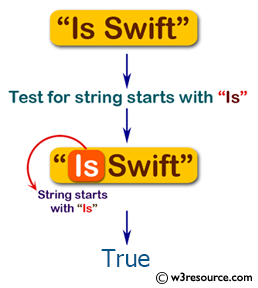﻿ Swift Basic Programming Exercise: Test a given string whether it starts with "Is" - w3resource# Swift Basic Programming Exercise: Test a given string whether it starts with "Is"

## Swift Basic Programming: Exercise-11 with Solution

Write a Swift program to test a given string whether it starts with "Is". Return true or false.

Pictorial Presentation:Sample Solution:

Swift Code:

``````func start_is(str1: String) -> Bool {
let str2 = str1.characters
let hello = "Is"
let first2Values = str2.prefix(2)
let first_two = String(first2Values)

if hello == first_two {
return true
} else {
return false
}
}

print(start_is(str1: "Is Swift"))
print(start_is(str1: "is python"))
print(start_is(str1: "java is"))
```
```

Sample Output:

```true
false
false
```

Swift Programming Code Editor:

Improve this sample solution and post your code through Disqus

What is the difficulty level of this exercise?

﻿